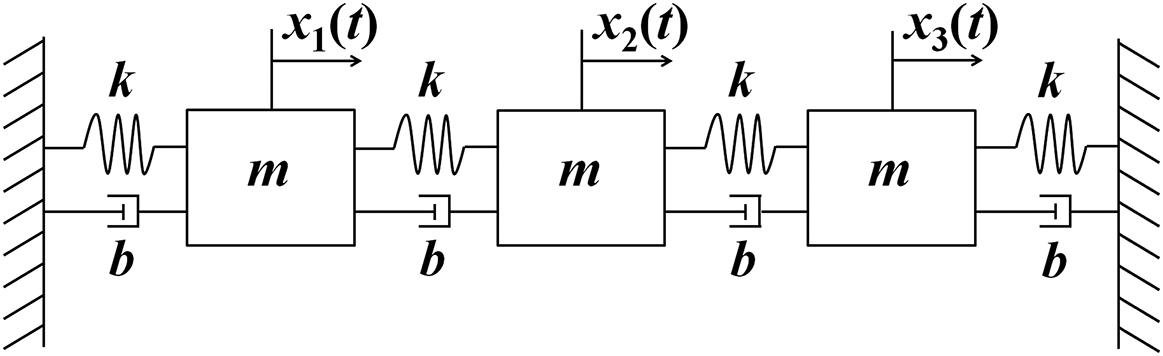#### Problem Statement:

Imagine a system where there are two masses of 1kg each that are between two walls. There is a spring attaching each mass to each wall and one connecting the two masses each of 10N/m. There is also a damping force of .01 N*s/m on each of the springs. Plot the displacement of each mass in two separate plots when a force of 0.8 rad/s acts on the first mass. The image below shows three masses, four springs, and four dampers, which can be done in the same code provided. Think of it as extra credit. Hint: Run the differential code when you run the main code.